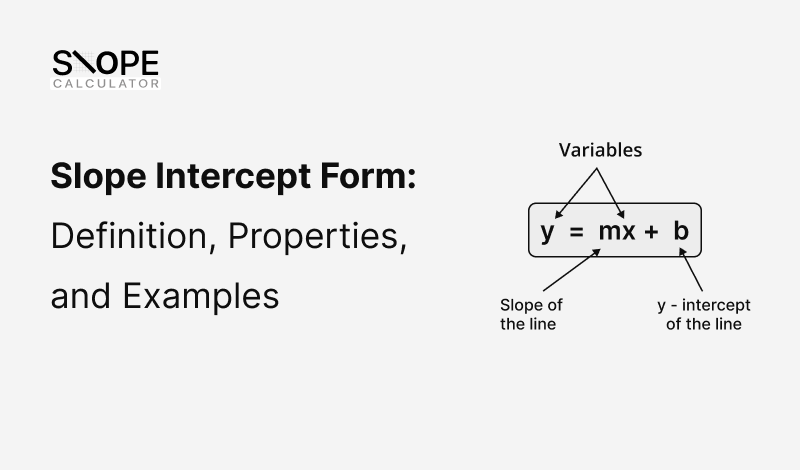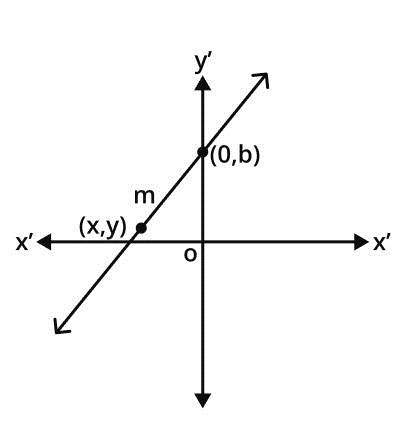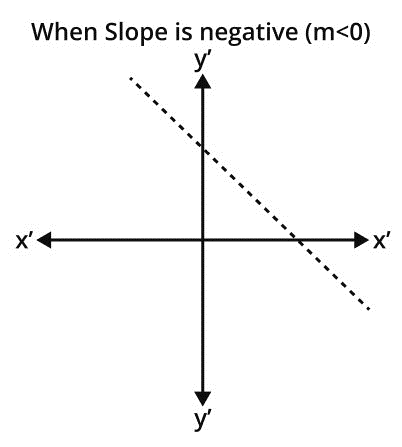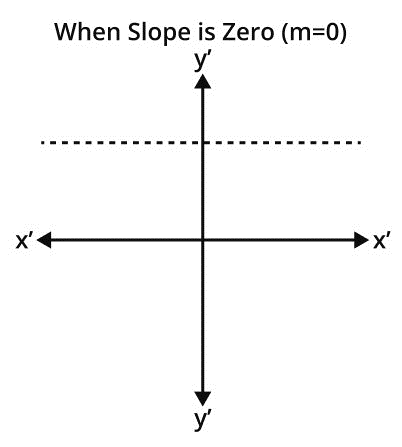## Slope Intercept Form: Definition, Properties, and ExamplesThe slope intercept form is a way of representing a linear equation in a two-dimensional coordinate plane. It defines the connection between two variables x and y in a straight line. This form is most useful when the slope and y-intercept of the line are known. The equation of a straight line may also be found using the slope-intercept form if two points on the line are given.

In this blog post, we’ll discuss the definition of slope intercept form and learn how to determine the equation of the line by using it along with examples.

## What is the Slope-Intercept Form of Line?

The slope intercept form is a particular and widely used form for writing the equation of a line. This form is highly effective in simplifying the representation and interpretation of straight-line equations. Slope intercept form requires the slope “m” and y-intercept “b” of the line to determine the equation of a line.

Assume that a line has slope m and y-intercept b. The slope-intercept form for linear equation with slope m and y-intercept b can be expressed as:

y = mx + b

Here m and b can be any real numbers. The value of m determines the inclination of a line while the value of b represents the point where the line crosses the y-axis.

## Equation of Slope - Intercept Form

Consider a straight line with slope m and intersects the y-axis at (0, b). Also (x, y) is any other arbitrary point on this line.The mathematical expression for the slope of a line passing through two points (x1, y1) and (x2, y2) is as follows:

Slope = m = (y2 – y1)/(x2 – x1)

Here

• (x1, y1) = (0, b)
• (x2, y2) = (x, y)

Put these values in the slope formula,

m = (y – b)/(x – 0)

m = (y – b)/(x)

Multiply ‘x’ on both sides.

mx = y – b

After rearranging the above equation, we get

y = mx + b

This is the general form of slope-intercept form.

## Formula of Slope intercept form

The formula for the slope-intercept form used to write the equation of a line is:

y = mx + b

Where,

• m denotes the slope or steepness of the line.
• (x, y) represent every point on a line.
• b is the y-intercept that indicates where the line cut the y-axis.

## Properties of Slope Intercept form

Some properties of slope intercept form are given here:

1. Slope intercept form is an easy and simple way to express the linear equation by using slope and y-intercept.
2. It is ideal for graphing linear equations because it directly gives the slope and y-intercept. This makes it easy to sketch the line on a graph.
3. This form is frequently used in real-world applications to represent relationships with a constant rate of change.
4. Understanding the slope and y-intercept helps in interpreting a line's behavior.
• For Positive Slope (m > 0): Line rises from left to right side.• If the slope is negative (m < 0): The line falls from left to right.• When the Slope is zero (m = 0): The line draws a horizontal parallel to the x-axis.## How to Determine Slope Intercept Form?

Let’s learn how to find the slope-intercept form in easy steps:

1. Find the slope (m) by the following formula.

Slope = m = (y2 – y1)/(x2 – x1)

Move on to the next step if the slope is already given.

1. Calculate the value of b (y-intercept)

The given point and slope m can be used to find b. Substitute the values of x, y, and m into the equation (y = mx + b) and calculate it for b.

Go to the next step if it is also given in question.

1. Once you have found the values of m and b, substitute them into the equation:

y = mx + b

Here are a few examples to clarify the above steps.

## Examples of Slope intercept form

Let's look at a couple of examples to see how slope intercept form works.

Example 1: (If Two Points are given)

Determine the equation of a straight line that passes through the points (2, 3) and (-1, 5).

Solution:

Here

(x₁, y₁) = (2, 3)

(x₂, y₂) = (-1, 5)

We will use the following formula to find the slope of this line.

m = (y₂ - y₁) / (x₂ - x₁)

m = (5 - 3) / (-1 - 2)

m = 2 / -3

Now that we have the slope, we can use the slope-intercept form to find b:

y = mx + b

y = (-2/3) x + b

As the line passes through the points (2, 3) we can substitute the values of x and y into the equation as follows:

3 = (-2/3) (2) + b

3 = -4/3 + b

b = 11/3

Substitute the value of b and m in the general equation of slope-intercept form.

y = (-2/3) x + 11/3

It is the equation that passes from (2, 3) and (-1, 5).

Example 2: (If Slope & y-intercept are given)

Find the equation of a straight line when the slope of a line is 3 and the y-intercept of the line is - 6.

Solution:

Here,

Slope = m = 3

Y-intercept = b = - 6

Substitute these values in the standard equation of the slope-intercept form.

y = mx + b

y = (3) x + (- 6)

y = 3x – 6

Example 3: (When 1 Point and Slope are known)

Determine the equation of a line that passes from the point (2, 5) with a slope of 3.

Solution:

To find the equation of this line, we can use the slope-intercept form as follows:

y = mx + b

y = 3x + b ______ (1)

We know that the line passes from the points (2, 5), so we can substitute the values of x and y into the equation as follows:

5 = 3(2) + b

5 = 6 + b

b = -1

Put the value of b in equation (1), we get

y = 3x – 1